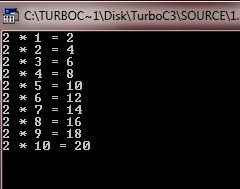# C goto Statement

In C language, the goto statement requires a label (a label is a valid identifier followed by a colon) for operation. Furthermore, the label must be in the same function as the goto that uses it, you can't jump between functions.

Here is the general form of the goto statement in C programming:

```label:
.
.
.
goto label;```

Here label is any valid label either before or after goto. Let's go through the following example program.

## C goto Statement Example

Here is an example program that uses the goto statement in C programming to illustrate it:

```/* C goto Statement Example
* This program illustrates the
* concept of goto statement in C
*/

#include<stdio.h>
#include<conio.h>
void main()
{
int i = 1;
int num = 2;
clrscr();

tab:
printf("%d * %d = %d\n", num, i, num*i);
i++;
if(i<=10)
goto tab;

getch();
}```

This program prints the table of 2. Here is the sample run of the above C program.Tools
Calculator